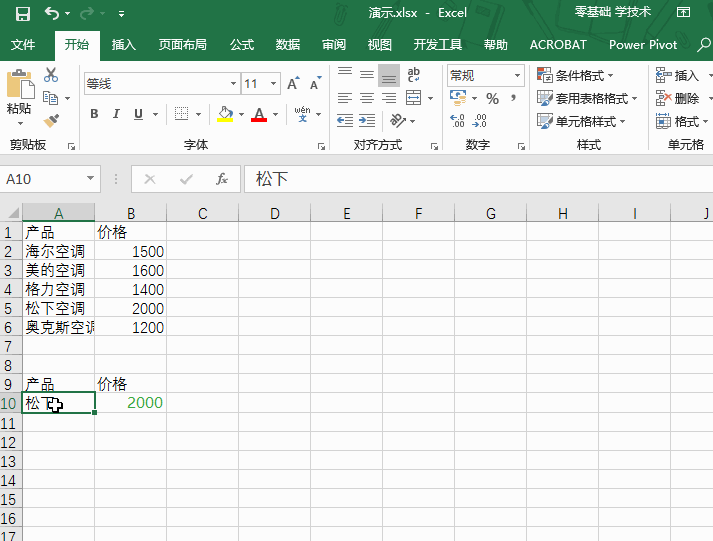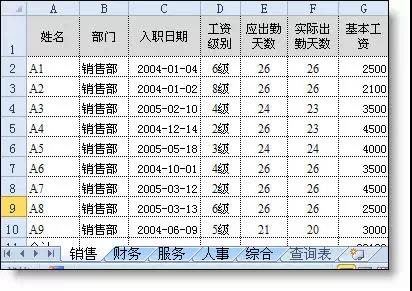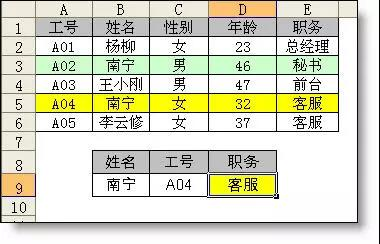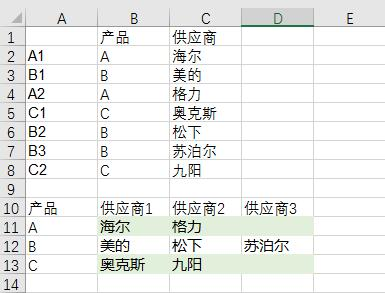# Vlookup函数5个高级用法

1、区间查找

=VLOOKUP(F3,\$B\$3:\$C\$11,2)2、模糊查找

=VLOOKUP("*"&A10&"*",A2:B6,2,0)3. 多条件查找

{=VLOOKUP(B9&C9,IF({1,0},B2:B6&A2:A6,E2:E6),2,0)}4、多工作表查找

=IFERROR(VLOOKUP(A2,服务!A:G,7,0),IFERROR(VLOOKUP(A2,人事!A:G,7,0),IFERROR(VLOOKUP(A2,综合!A:G,7,0),IFERROR(VLOOKUP(A2,财务!A:G,7,0),IFERROR(VLOOKUP(A2,销售!A:G,7,0),"无此人信息")))))

=VLOOKUP(A2,INDIRECT(LOOKUP(1,0/COUNTIF(INDIRECT({"销售";"服务";"人事";"综合";"财务"}&"!a:a"),A2),{"销售";"服务";"人事";"综合";"财务"})&"!a:g"),7,0)5、一对多查找

A2 =B2&COUNTIF(B\$1:B2,B2)

B11=IFERROR(VLOOKUP(\$A11&COLUMN(A1),\$A:\$C,3,0),"")